# Primary maths: Fractions

Resources team
10th January 2020## Ensure your students aren't frazzled by fractions with these stimulating and straightforward resources

Sometimes the best way to overcome any negativity or concern for a topic like fractions is by adding a handful of fresh ideas to your toolbox. With that in mind, we've picked out just some of the varied ways that the Tes community have chosen to tackle the challenge head on. Why not take a look at what they've come up with?

### KS1#### Shading fractions of a shape

This is a worksheet for colouring fractions of shapes. Featuring 1/4, 1/2, 3/4 and 1/3.#### Fractions for Year 1

These were some sheets I designed for our introduction to fractions. We had already looked at doubling and halving and next was halves, quarters and three quarters of shapes and numbers. These are differentiated worksheets made for Year 1.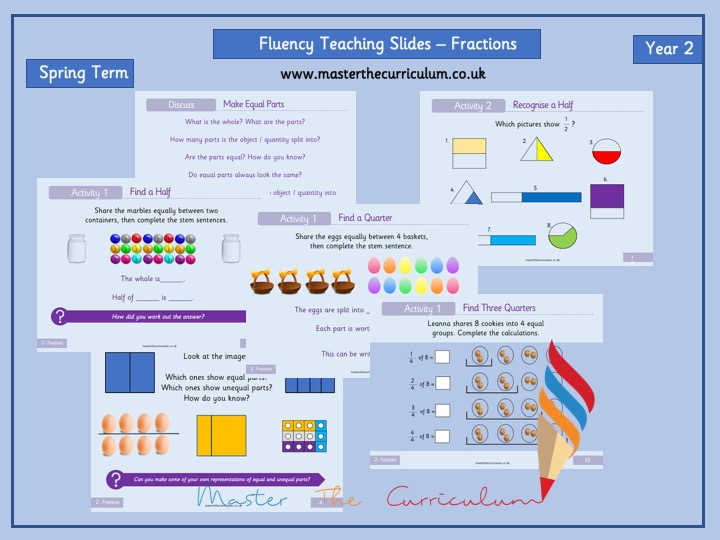#### Year 2 - Fractions White Rose Style Fluency Teaching Slides

Updated to reflect the New White Rose Plans.

These teaching slides are useful for those following White Rose.

The fractions unit is for Year 2, Spring Term, Block 4.#### Y1 planning and resources for White Rose Maths Summer Block 2 Fractions week 1

Hello again. This is my first week of planning and resources to go with the White Rose Maths scheme of learning for Year 1, Summer Block 2, Fractions. It is in my usual format with flipcharts in activprimary and smartboard and there is also a PDF version too. All the other resources are in word so that you can adapt them for your children. I’ve tried to keep up the problem solving this week but I think, with adult, concrete and visual support, modelling, discussion and the small steps, they’ll work well. As always, I hope they save you some time and that your children enjoy them.#### Year 2 Fractions Assessment

Year 2 Fractions Assessment that covers:
* Identifying fractions of shapes
* Finding 1/2 and 1/4s of a shape
* Finding 1/2 and 1/4 of a quantity
* Finding 1/2 and 1/4 of a whole number
* Identifying equivalent fractions

There is a checklist for teacher or child to tick what they can do. I also highlight each one the child meets to make it stand out. It is an assessment scored out of 11. It is great way to check where the child is and see who needs intervention work on fractions.#### Fraction Word Problems

Differentiated three ways, using halves, quarters and thirds. Real life word problems, using weight and money. 1 and 2 step word problems, varying in difficulty.

### Lower KS2#### Equivalent Fractions - Year 4

This worksheet was created by primaryleap.co.uk

We can make equivalent fractions by multiplying or dividing both top and bottom by the same amount. Students are asked to complete the equivalent fractions.#### Adding fractions with the same denominator.

Various differentiated worksheets aimed at getting children to understand how to add fractions, that have the same denominator, to make a whole one. Fraction PowerPoint to recap the basics of fractions.#### Year 3 Fraction Word Problems

Differentiated fraction word problems for year 3.
Problems involve single step and multi-step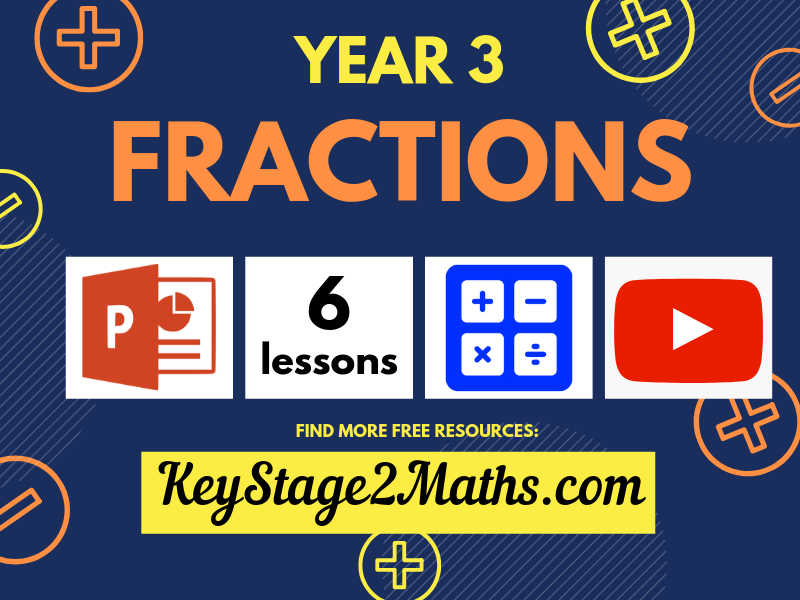#### Year 3 | Fractions

Lessons included: Recognise and name unit and non-unit fractions; Compare and order fractions (with either the same denominator or same numerator); Count on and back in unitary fractions; Add and subtract fractions with the same denominator; Solve missing number problems for addition and subtraction with fractions; Find a fraction of a number (for unitary fractions)#### Differentiated fractions worksheets

Some of my mixed 3/4 class struggled with finding fractions where the numerator was higher than 1 (e.g. 2/3 of 15). They also really struggled with putting them in word problems! I created these step by step worksheets so children could see the progression, e.g. 1/3 of 15 is? 2/3 of 15 is? I have 15 sweets and I eat 2/3 of them. How many do I eat?#### Year 4 – Spring Block 3 – Fractions - week 6 - Count in fractions

This resource follows the concrete-pictorial-abstract approach to Maths Mastery and also includes reasoning questions. It is differentiated two ways and linked to WRM small step: Count in fractions.

- Recognise and show, using diagrams, families of common equivalent fractions.

I hope you find this resource useful,

Your feedback is always welcome :)

### Upper KS2#### Improper fractions and mixed numbers

- PPT introducing topic in basic manner. - Worksheet where pupils must identify the mixed numbers and improper fractions.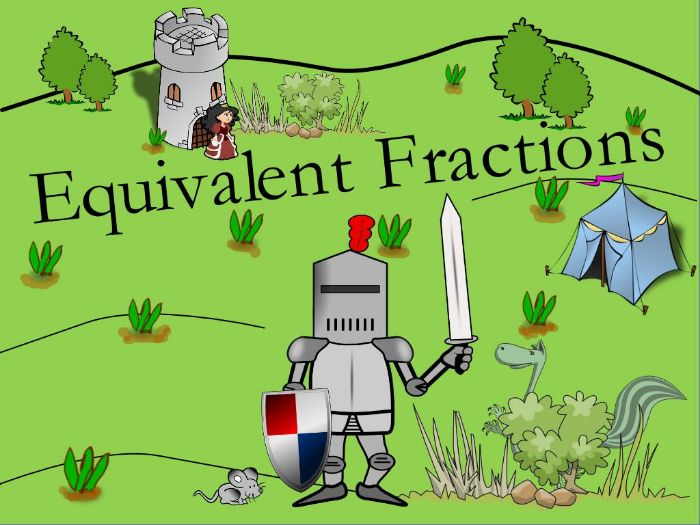#### KS2 Equivalent Fractions Resource Pack

This is a complete resource pack for teaching equivalent fractions objectives in KS2. Included is a 62 page flip-chart (I have uploaded in ActivInspire, PowerPoint and PDF format) to help deliver the lessons, a 46 page booklet of differentiated activities including puzzles, games and worksheets. I have also included a 5 page planning tool so that you can copy and paste plans into your own planning format and adjust as you wish for each activity. Furthermore, I have included Word versions so you can adapt all activities to your own pupil’s needs if required. This pack will save you hours and hours and hours of planning and generating worksheets, games, problem solving activities and flip-charts. Children will love the themed activities and the great characters in this resource pack and it will make learning about equivalent fractions fun!

Objectives covered:

Recognise and show, using diagrams, equivalent fractions with small denominators
Recognise and show, using diagrams, families of common equivalent fractions
Identify, name and write equivalent fractions of a given fraction, represented visually, including tenths and hundredths
Use common factors to simplify fractions

More like this…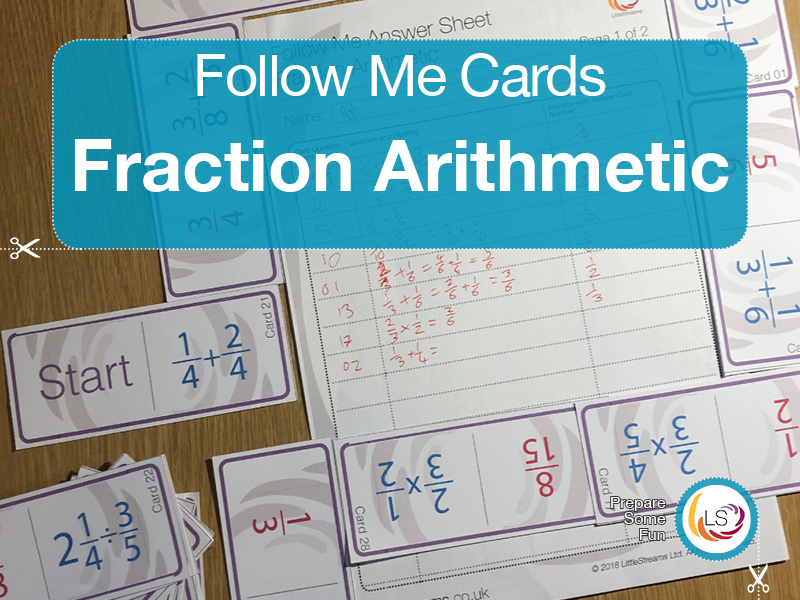#### Fractions Arithmetic | Follow Me Cards

This pack contains 30 follow me cards on adding and multiplying fractions. Early cards include adding and subtracting fractions with the same denominators or where only one fraction needs to be made into an equivalent. Questions move on to adding fractions with different denominators, multiplying and dividing fractions including where cross cancelling is expected.

There are two possible starting cards to allow for differentiation.

These 30 follow me cards are a great way to revise arithmetic with fractions. Play is similar to dominoes, where the cards are placed end to end with the question to the answers, but each question only has one answer. Students connect the questions and answers together forming a chain, with questions steadily getting harder.

Starting with addition of fractions with the same denominators, the questions quickly progress to addition and subtraction with di erent denominators, multiplication and division with fractions, including mixed numbers.

Some ideas for how to use follow me cards:

-Have students race to connect the cards.

-Have a treasure hunt, around the classroom or outside. Students start with the starter card and record the solutions in the table.

-Students each take ownership of a card, and then get themselves in line correctly with the answers. If there are more than 30 students, assign some students as ‘managers’. If there are less than 30 students, the first students to connect their cards can be given the remaining cards.

- Cut each card in half and give the students a matching activity. They can then glue the answers into their books.

You can get this as part of the bundle at a discount:
https://www.tes.com/teaching-resource/fraction-and-percentage-follow-me-card-bundle-12129689.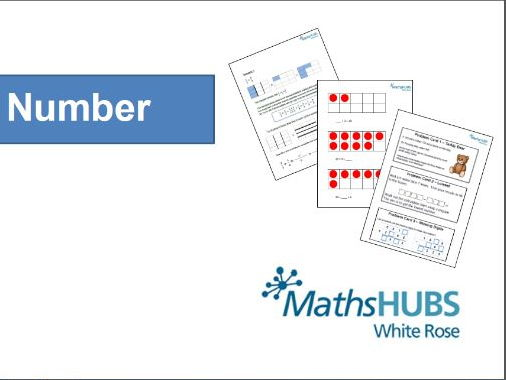#### Mixed numbers & improper fractions

This selection of resources will help children to embed their understanding of converting between mixed numbers and improper fractions.#### Multiplying fractions by a whole number KS2

A power point introduction and three differentiated activity sheets for multiplying fractions by a whole number. The challenge level 3 also contains mixed number fractions multiplied by a whole number. Please rate and leave a comment about how you get on with it!#### Maths Murder Mystery (KS2 Fractions)

Maths Murder Mystery (KS2 Fractions)

Review fractions with this fun mini murder mystery.
Great for revising, practising fractions of amounts.
Included a worded problem, fractions of amounts, fractions with clocks/ money and on a number line.

Te resource contains 3 pages - 2 with the murder mystery, 1 with answers and HoppyTimes terms and conditions.
Thank you for your interest in my products!